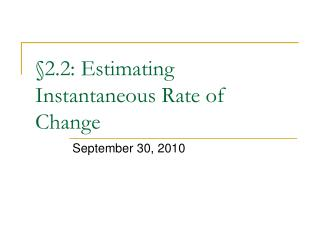# §2.2: Estimating Instantaneous Rate of Change - PowerPoint PPT PresentationDownload Presentation§2.2: Estimating Instantaneous Rate of Change

§2.2: Estimating Instantaneous Rate of ChangeDownload Presentation## §2.2: Estimating Instantaneous Rate of Change

- - - - - - - - - - - - - - - - - - - - - - - - - - - E N D - - - - - - - - - - - - - - - - - - - - - - - - - - -
##### Presentation Transcript

1. §2.2: Estimating Instantaneous Rate of Change September 30, 2010

2. Review of AROC

3. Review of AROC • graphically related to the secant line intersecting the graph of a function at two points

4. Difference Quotient • basically the same as delta x over delta y but combines the concept the delta concept with function notation • ∆x is the size of our interval and we replace that expression with h

5. Instantaneous Rate of Change • we estimate the IROC of a function f(x) at a point x = a by examining the AROC with a very small interval around the value of x = a • represented graphically by a tangent line to the curve f(x) at the point x = a

6. Tangent Line • a line that intersectsthe curve at asingle point

7. Interval Method of Estimating IROC • to estimate the IROC of a function at a point, we need to first talk about the intervals we can use…

8. Intervals • preceding interval • an interval having an upper bound as the value of x in which we are interested • following interval • an interval having a lower bound as the value of x in which we are interested

9. Intervals (cont.) • centred interval • an interval containing the value of x in which we are interested

10. Method for Determining IROC • easiest way is with a centred interval • you must “look” on both sides of the point • two successive approximations • one is insufficient, you are looking for convergence • we want our ∆x or “h” to be as small as possible, (∆x < 0.1 is usually safe) • at least on the second approximation • want to see if the difference quotient gets closer to a certain value as the size of the interval becomes smaller, 3 successive approximations allows us to perform more careful trend analysis

11. Graphically…

12. Example • Determine the IROC of f(x) = x2 + 1 at x=2

13. Difference between AROC and IROC • AROC → over an interval • IROC → at a point • although technically IROC is an estimation in this course so it is over a small interval as an approximation to a point

14. Advanced Algebraic Method • doesn’t use actual numerical values of h or ∆x for the interval but is based on the idea that the size of the interval becomes infinitely small in size • requires solid algebraic skills • relies on the difference quotient definition • allows you to calculate the exact IROC at a point and avoid an estimation

15. Example • Determine the exact IROC of f(x) = x2 + 1 at x=2

16. What do I need to know? • you MUST be able to estimate the instantaneous rate of change of a function at a point via the method of successive approximations

17. Homework • §2.2 p.85 #1-4, 6, 7, 9, 10, 12, 15 • Reading p.89-91